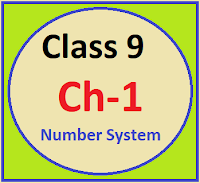## Posts

Showing posts from April, 2022

### Common Errors in Secondary Mathematics

Common Errors Committed  by the  Students  in Secondary Mathematics   Errors  that students often make in doing secondary mathematics  during their practice and during the examinations  and their remedial measures are well explained here stp by step.  Some Common Errors in Mathematics

### NCERT Solution Class 9 Ch-1 Number SystemClass 9 Chapter - 1 Number System Numbers are the backbone of mathematics. Without numbers mathematics looks impossible. So students should have complete knowledge of number system. Before start this chapter students should go through some key points which are very important for accelerating the things. BEFORE START NCERT CHAPTER 1 CLASS IX, STUDENTS SHOULD LEARN THE FOLLOWINGS Tables (2-20), Square tables (1-30), Cubic tables (1-20) Complete number system with definitions . Different types of decimals . METHOD OF FINDING RATIONAL NUMBERS BETWEEN GIVEN TWO NUMBERS. Let two numbers are 2 and 3 These numbers can be written as 2.0 and 3.0 Rational numbers between 2 and 3 are as follows 2.1,  2.2,  2.3,  2.4,  2.5,  2.6,  2.7,  2.8,  2.9 etc. When two fractions are given then algorithm to find the rational numbers between two fractions Algorithm 1) Make the denominator of both the fractions equal by taking their LCM